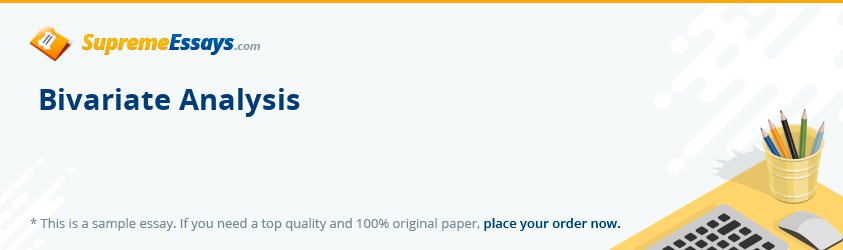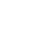Live chatBivariate analysis is a simple form of quantitative analysis that involves analysis between two variables. The main reason for this is to determine the existence of the empirical relationship among them. This will show the difference between variables or their strength. Bivariate analysis can be conducted using a variety of ways. The most common and the simplest analysis technique is the use of graphs. The graphs include box plots and 3-D Bar graphs. In addition, there are numerical methods like cross tabulations with Chi squire and one-way ANOVA analysis. A 3-D Bar graph is used when showing the relationship existing between a pair of categorical variables. A scatter plot analyses a pair of continuous data and box plot shows the relationship between a category and a continuous variable.

A T-test is a statistical examination of a mean of two populations. It looks at the t - distribution, t- statistic plus a freedom degree to find if the populations mean differ. Often scientific research examines the nature’s phenomena a pair of variables at the same time ready to answer questions like are they related and what will be the effect of varying the level of one and many more. Here is an example of a variable that can be tested using a T-test. When experimenting if the growth of a particular type of plant can be caused by the presence of a certain of a certain type of mycorrhizal fungus.Chi-square Test is used in comparing observed data expected to be obtained due to a certain hypothesis. An example can be obtained from Mendel’s law. In an investigation to determine the parental genotype of a cross between two pea plants with a plant population yield of 870 plants, 629 green seeded and 241 yellow seeded. With a Chi-Squire Test, it is ease to find the answer.

Correlation is the measure of the associated between two variables. This is normally calculated in randomly selected variables. An example is the correlation between the salary earned and the wealth accumulated. Both of these variables are continuous and are expressed in monetary terms.

0

Preparing Orders

0

Active Writers

0%

Positive Feedback

0

Support Agents

Now Accepting Apple Pay!
Limited offerget 15% off your 1st order with code first15